1678051620

# A Practical Guide of Unsupervised Learning Algorithms

In this article, learn about Machine Learning Tutorial: A Practical  Guide of Unsupervised Learning Algorithms. Machine learning is a fast-growing technology that allows computers to learn from the past and predict the future. It uses numerous algorithms for building mathematical models and predicting future trends. Machine learning (ML) has widespread applications in the industry, including speech recognition, image recognition, churn prediction, email filtering, chatbot development, recommender systems, and much more.

Machine learning (ML) can be classified into three main categories; supervised, unsupervised, and reinforcement learning. In supervised learning, the model is trained on labeled data. While in unsupervised learning, unlabeled data is provided to the model to predict the outcomes. Reinforcement learning is feedback learning in which the agent collects a reward for each correct action and gets a penalty for a wrong decision. The goal of the learning agent is to get maximum reward points and deduce the error.

## What is Unsupervised Learning?

In unsupervised learning, the model learns from unlabeled data without proper supervision.

Unsupervised learning uses machine learning techniques to cluster unlabeled data based on similarities and differences. The unsupervised algorithms discover hidden patterns in data without human supervision. Unsupervised learning aims to arrange the raw data into new features or groups together with similar patterns of data.

For instance, to predict the churn rate, we provide unlabeled data to our model for prediction. There is no information given that customers have churned or not. The model will analyze the data and find hidden patterns to categorize into two clusters: churned and non-churned customers.## Unsupervised Learning Approaches

Unsupervised algorithms can be used for three tasks—clustering, dimensionality reduction, and association. Below, we will highlight some commonly used clustering and association algorithms.

#### Clustering Techniques

Clustering, or cluster analysis, is a popular data mining technique for unsupervised learning. The clustering approach works to group non-labeled data based on similarities and differences. Unlike supervised learning, clustering algorithms discover natural groupings in data.

A good clustering method produces high-quality clusters having high intra-class similarity (similar data within a cluster) and less intra-class similarity (cluster data is dissimilar to other clusters).

It can be defined as the grouping of data points into various clusters containing similar data points. The same objects remain in the group that has fewer similarities with other groups. Here, we will discuss two popular clustering techniques: K-Means clustering and DBScan Clustering.

#### K-Means Clustering

K-Means is the simplest unsupervised technique used to solve clustering problems. It groups the unlabeled data into various clusters. The K value defines the number of clusters you need to tell the system how many to create.

K-Means is a centroid-based algorithm in which each cluster is associated with the centroid. The goal is to minimize the sum of the distances between the data points and their corresponding clusters.

It is an iterative approach that breaks down the unlabeled data into different clusters so that each data point belongs to a group with similar characteristics.

1. Using an iterative process to create the best value of K.
2. Each data point is assigned to its closest k-center. The data point that is closer to the particular k-center makes a cluster.An illustration of K-means clustering. Image source

#### DBScan Clustering

“DBScan” stands for “Density-based spatial clustering of applications with noise.” There are three main words in DBscan: density, clustering, and noise. Therefore, this algorithm uses the notion of density-based clustering to form clusters and detect the noise.

Clusters are usually dense regions that are separated by lower density regions. Unlike the k-means algorithm, which works only on well-separated clusters, DBscan has a wider scope and can create clusters within the cluster. It discovers clusters of various shapes and sizes from a large set of data, which consists of noise and outliers.

There are two parameters in the DBScan algorithm:

minPts: The threshold, or the minimum number of points grouped together for a region considered as a dense region.

eps(ε): The distance measure used to locate the points in the neighborhood.An illustration of density-based clustering. Image Source

### Association Rule Mining

An association rule mining is a popular data mining technique. It finds interesting correlations in large numbers of data items. This rule shows how frequently items occur in a transaction.

Market Basket Analysis is a typical example of an association rule mining that finds relationships between items in the grocery store. It enables retailers to identify and analyze the associations between items that people frequently buy.

Important terminology used in association rules:

Support: It tells us about the combination of items bought frequently or frequently bought items.

Confidence: It tells us how often the items A and B occur together, given the number of times A occurs.

Lift: The lift indicates the strength of a rule over the random occurrence of A and B. For instance, A->B, the life value is 5. It means that if you buy A, the occurrence of buying B is five times.

The Apriori algorithm is a well-known association rule based technique.

#### Apriori algorithm

The Apriori algorithm was proposed by R. Agarwal and R. Srikant in 1994 to find the frequent items in the dataset. The algorithm’s name is based on the fact that it uses prior knowledge of frequently occurring things.

The Apriori algorithm finds frequently occurring items with minimum support.

It consists of two steps:

• Generation of candidate itemsets.
• Removing items that are infrequent and don’t fulfill the criteria of minimum support.

## Practical Implementation of Unsupervised Algorithms

In this tutorial, you will learn about the implementation of various unsupervised algorithms in Python. Scikit-learn is a powerful Python library widely used for various unsupervised learning tasks. It is an open-source library that provides numerous robust algorithms, which include classification, dimensionality reduction, clustering techniques, and association rules.

Let’s begin!

### 1. K-Means algorithm

Now let’s dive deep into the implementation of the K-Means algorithm in Python. We’ll break down each code snippet so that you can understand it easily.

#### Import libraries

First of all, we will import the required libraries and get access to the functions.

``````#Let's import the required libraries
import numpy as np
import pandas as pd
import matplotlib.pyplot as plt
import seaborn as sns``````

The dataset is taken from the kaggle website. You can easily download it from the given link. To load the dataset, we use the pd.read_csv() function. head() returns the first five rows of the dataset.

my_data = pd.read_csv('Customers_Mall.csv.') my_data.head()The dataset contains five columns: customer ID, gender, age, annual income in (K\$), and spending score from 1-100.

#### Data Preprocessing

The info() function is used to get quick information about the dataset. It shows the number of entries, columns, total non-null values, memory usage, and datatypes.

my_data.info()To check the missing values in the dataset, we use isnull().sum(), which returns the total number of null values.

``````#Check missing values
my_data.isnull().sum()``````The box plot or whisker plot is used to detect outliers in the dataset. It also shows a statistical five number summary, which includes the minimum, first quartile, median (2nd quartile), third quartile, and maximum.

my_data.boxplot(figsize=(8,4))Using Box Plot, we’ve detected an outlier in the annual income column. Now we will try to remove it before training our model.

``````#let's remove outlier from data
med =61
my_data["Annual Income (k\$)"] = np.where(my_data["Annual Income (k\$)"] >
120,med,my_data['Annual Income (k\$)'])

``````

The outlier in the annual income column has been removed now to confirm we used the box plot again.

my_data.boxplot(figsize=(8,5))#### Data Visualization

A histogram is used to illustrate the important features of the distribution of data. The hist() function is used to show the distribution of data in each numerical column.

my_data.hist(figsize=(6,6))

The correlation heatmap is used to find the potential relationships between variables in the data and to display the strength of those relationships. To display the heatmap, we have used the seaborn plotting library.

plt.figure(figsize=(10,6)) sns.heatmap(my_data.corr(), annot=True, cmap='icefire').set_title('seaborn') plt.show()#### Choosing the Best K Value

The iloc() function is used to select a particular cell of the data. It enables us to select a value that belongs to a specific row or column. Here, we’ve chosen the annual income and spending score columns.

X_val = my_data.iloc[:, 3:].values X_val``````# Loading Kmeans Library

from sklearn.cluster import KMeans``````

Now we will select the best value for K using the Elbow’s method. It is used to determine the optimal number of clusters in K-means clustering.

``````my_val = []

for i in range(1,11):

kmeans = KMeans(n_clusters = i, init='k-means++', random_state = 123)

kmeans.fit(X_val)

my_val.append(kmeans.inertia_)``````

The sklearn.cluster.KMeans() is used to choose the number of clusters along with the initialization of other parameters. To display the result, just call the variable.

my_val#Visualization of clusters using elbow’s method plt.plot(range(1,11),my_val) plt.xlabel('The No of clusters') plt.ylabel('Outcome') plt.title('The Elbow Method') plt.show()Through Elbow’s Method, when the graph looks like an arm, then the elbow on the arm is the best value of K. In this case, we’ve taken K=3, which is the optimal value for K.

kmeans = KMeans(n_clusters = 3, init='k-means++') kmeans.fit(X_val)#To show centroids of clusters  kmeans.cluster_centers_#Prediction of K-Means clustering  y_kmeans = kmeans.fit_predict(X_val) y_kmeans

#### Splitting the dataset into three clusters

The scatter graph is used to plot the classification results of our dataset into three clusters.

``````plt.scatter(X_val[y_kmeans == 0,0], X_val[y_kmeans == 0,1], c='red',s=100)

plt.scatter(X_val[y_kmeans == 1,0], X_val[y_kmeans == 1,1], c='green',s=100)

plt.scatter(X_val[y_kmeans == 2,0], X_val[y_kmeans == 2,1], c='orange',s=100)

plt.scatter(kmeans.cluster_centers_[:,0], kmeans.cluster_centers_[:,1], s=300, c='brown')

plt.title('K-Means Unsupervised Learning')

plt.show()

``````

### 2. Apriori Algorithm

To implement the apriori algorithm, we will utilize “The Bread Basket” dataset. The dataset is available on Kaggle and you can download it from the link. This algorithm suggests products based on the user’s purchase history. Walmart has greatly utilized the algorithm to recommend relevant items to its users.

Let’s implement the Apriori algorithm in Python.

#### Import libraries

To implement the algorithm, we need to import some important libraries.

``````import pandas as pd

import matplotlib.pyplot as plt

import numpy as np

import seaborn as sns``````

The dataset contains five columns and 20507 entries. The data_time is a prominent column and we can extract many vital insights from it.

my_data= pd.read_csv("bread basket.csv") my_data.head()#### Data Preprocessing

Convert the data_time into an appropriate format.

``````my_data['date_time'] = pd.to_datetime(my_data['date_time'])

#Total No of unique customers

my_data['Transaction'].nunique()``````

Now we want to extract new columns from the data_time to extract meaningful information from the data.

``````#Let's extract date

my_data['date'] = my_data['date_time'].dt.date

#Let's extract time

my_data['time'] = my_data['date_time'].dt.time

#Extract month and replacing it with String

my_data['month'] = my_data['date_time'].dt.month

my_data['month'] = my_data['month'].replace((1,2,3,4,5,6,7,8,9,10,11,12),

('Jan','Feb','Mar','Apr','May','Jun','Jul','Aug',

'Sep','Oct','Nov','Dec'))``````

#Extract hour

my_data[‘hour’] = my_data[‘date_time’].dt.hour

# Replacing hours with text

# Replacing hours with text

hr_num = (1,7,8,9,10,11,12,13,14,15,16,17,18,19,20,21,22,23)

hr_obj = (‘1-2′,’7-8′,’8-9′,’9-10′,’10-11′,’11-12′,’12-13′,’13-14′,’14-15’,

’15-16′,’16-17′,’17-18′,’18-19′,’19-20′,’20-21′,’21-22′,’22-23′,’23-24′)

my_data[‘hour’] = my_data[‘hour’].replace(hr_num, hr_obj)

# Extracting weekday and replacing it with String

my_data[‘weekday’] = my_data[‘date_time’].dt.weekday

my_data[‘weekday’] = my_data[‘weekday’].replace((0,1,2,3,4,5,6),

(‘Mon’,’Tues’,’Wed’,’Thur’,’Fri’,’Sat’,’Sun’))

#Now drop date_time column

my_data.drop(‘date_time’, axis = 1, inplace = True)

After extracting the date, time, month, and hour columns, we dropped the data_time column.

Now to display, we simply use the head() function to see the changes in the dataset.# cleaning the item column

my_data[‘Item’] = my_data[‘Item’].str.strip()

my_data[‘Item’] = my_data[‘Item’].str.lower()#### Data Visualization

To display the top 10 items purchased by customers, we used a barplot() of the seaborn library.

``````plt.figure(figsize=(10,5))

plt.xlabel('No of Items', size = 17)

plt.xticks(rotation=45)

plt.ylabel('Total Items', size = 18)

plt.title('Top 10 Items purchased', color = 'blue', size = 23)

plt.show()

``````

From the graph, coffee is the top item purchased by the customers, followed by bread.

Now, to display the number of orders received each month, the groupby() function is used along with barplot() to visually show the results.

mon_Tran =my_data.groupby('month')['Transaction'].count().reset_index() mon_Tran.loc[:,"mon_order"] =[4,8,12,2,1,7,6,3,5,11,10,9] mon_Tran.sort_values("mon_order",inplace=True) plt.figure(figsize=(12,5)) sns.barplot(data = mon_Tran, x = "month", y = "Transaction") plt.xlabel('Months', size = 14) plt.ylabel('Monthly Orders', size = 14) plt.title('No of orders received each month', color = 'blue', size = 18) plt.show()To show the number of orders received each day, we applied groupby() to the weekday column.

``````wk_Tran = my_data.groupby('weekday')['Transaction'].count().reset_index()

wk_Tran.loc[:,"wk_ord"] = [4,0,5,6,3,1,2]

wk_Tran.sort_values("wk_ord",inplace=True)

plt.figure(figsize=(11,4))

sns.barplot(data = wk_Tran, x = "weekday", y = "Transaction",palette='RdYlGn')

plt.xlabel('Week Day', size = 14)

plt.ylabel('Per day orders', size = 14)

plt.title('Orders received per day', color = 'blue', size = 18)

plt.show()

``````

#### Implementation of the Apriori Algorithm

We import the mlxtend library to implement the association rules and count the number of items.

``````from mlxtend.frequent_patterns import association_rules, apriori

tran_str= my_data.groupby(['Transaction', 'Item'])['Item'].count().reset_index(name ='Count')

Now we’ll make a mxn matrix where m=transaction and n=items, and each row represents whether the item was in the transaction or not.

``````Mar_baskt = tran_str.pivot_table(index='Transaction', columns='Item', values='Count', aggfunc='sum').fillna(0)

We want to make a function that returns 0 and 1. 0 means that the item wasn’t present in the transaction, while 1 means the item exists.

``````def encode(val):

if val<=0:

return 0

if val>=1:

return 1

#Let's apply the function to the dataset

#using apriori algorithm to set min_support 0.01 means 1% freq_items = apriori(Basket, min_support = 0.01,use_colnames = True) freq_items.head()

Using the association_rules() function to generate the most frequent items from the dataset.

App_rule= association_rules(freq_items, metric = "lift", min_threshold = 1) App_rule.sort_values('confidence', ascending = False, inplace = True) App_rule.head()From the above implementation, the most frequent items are coffee and toast, both having a lift value of 1.47 and a confidence value of 0.70.

### 3. Principal Component Analysis

Principal component analysis (PCA) is one of the most widely used unsupervised learning techniques. It can be used for various tasks, including dimensionality reduction, information compression, exploratory data analysis and Data de-noising.

Let’s use the PCA algorithm!

First we import the required libraries to implement this algorithm.

``````import numpy as np

import pandas as pd

import matplotlib.pyplot as plt

import seaborn as sns

%matplotlib inline

from sklearn.decomposition import PCA

To implement the PCA algorithm the load_digits dataset of Scikit-learn is used which can easily be loaded using the below command. The dataset contains images data which include 1797 entries and 64 columns.

``````#Load the dataset

#Creating features

X_value = my_data.data

#Creating target

#Let's check the shape of X_value

X_value.shape``````#Each image is 8x8 pixels therefore 64px  my_data.images#Let's display the image plt.gray()  plt.matshow(my_data.images)  plt.show()Now let’s project data from 64 columns to 16 to show how 16 dimensions classify the data.

``````X_val = my_data.data

y_val = my_data.target

my_pca = PCA(16)

X_projection = my_pca.fit_transform(X_val)

print(X_val.shape)

print(X_projection.shape)``````

Using colormap we visualize that with only ten dimensions  we can classify the data points. Now we’ll select the optimal number of dimensions (principal components) by which data can be reduced into lower dimensions.

``````plt.scatter(X_projection[:, 0], X_projection[:, 1], c=y_val, edgecolor='white',

cmap=plt.cm.get_cmap("gist_heat",12))

plt.colorbar();``````
``````pca=PCA().fit(X_val)

plt.plot(np.cumsum(my_pca.explained_variance_ratio_))

plt.xlabel('Principal components')

plt.ylabel('Explained variance')

Based on the below graph, only 12 components are required to explain more than 80% of the variance which is still better than computing all the 64 features. Thus, we’ve reduced the large number of dimensions into 12 dimensions to avoid the dimensionality curse. pca=PCA().fit(X_val)

plt.plot(np.cumsum(pca.explained_variance_ratio_))

plt.xlabel('Principal components')

plt.ylabel('Explained variance')

#Let's visualize how it looks like

Unsupervised_pca = PCA(12)

X_pro = Unsupervised_pca.fit_transform(X_val)

print("New Data Shape is =>",X_pro.shape)

#Let's Create a scatter plot

plt.scatter(X_pro[:, 0], X_pro[:, 1], c=y_val, edgecolor='white',

cmap=plt.cm.get_cmap("nipy_spectral",10))

plt.colorbar();

``````

## Wrapping UpIn this machine learning tutorial, we’ve implemented the Kmeans, Apriori, and PCA algorithms. These are some of the most widely used algorithms, having numerous industrial applications and solve many real world problems. For instance, K-means clustering is used in astronomy to study stellar and galaxy spectra, solar polarization spectra, and X-ray spectra. And, Apriori is used by retail stores to optimize their product inventory.

Dreaming of becoming a data scientist or data analyst even without a university and a college degree? Do you need the knowledge of data science and analysis for promotions in your current role?

Are you interested in securing your dream job in data science and analysis and looking for a way to get started, we can help you? With over 10 years of experience in data science and data analysis, we will teach you the rubrics, guiding you with one-on-one lessons from the fundamentals until you become a pro.

Our courses are affordable and easy to understand with numerous exercises and assignments you can learn from. At the completion of our courses, you’ll be readily equipped with technical and practical skills to take on any data science and data analysis role in companies, collaborate effectively among teams and help businesses meet and exceed their objectives by extracting actionable insights from data.

Original article sourced at: https://thedatascientist.com

#machine-learning

## Buddha Community1604091840

## Supervised Learning vs Unsupervised Learning

Note from Towards Data Science’s editors:_ While we allow independent authors to publish articles in accordance with our rules and guidelines, we do not endorse each author’s contribution. You should not rely on an author’s works without seeking professional advice. See our Reader Terms for details._

Nowadays, nearly everything in our lives can be quantified by data. Whether it involves search engine results, social media usage, weather trackers, cars, or sports, data is always being collected to enhance our quality of life. How do we get from all this raw data to improve the level of performance? This article will introduce us to the tools and techniques developed to make sense of unstructured data and discover hidden patterns. Specifically, the main topics that are covered are:

1. Supervised & Unsupervised Learning and the main techniques corresponding to each one (Classification and Clustering, respectively).

2. An in-depth look at the K-Means algorithm

Goals

1. Understanding the many different techniques used to discover patterns in a set of data

2. In-depth understanding of the K-Means algorithm

## 1.1 Unsupervised and supervised learning

In unsupervised learning, we are trying to discover hidden patterns in data, when we don’t have any labels. We will go through what hidden patterns are and what labels are, and we will go through real data examples.

What is unsupervised learning?

First, let’s step back to what learning even means. In machine learning in statistics, we are typically trying to find hidden patterns in data. Ideally, we want these hidden patterns to help us in some way. For instance, to help us understand some scientific results, to improve our user experience, or to help us maximize profit in some investment. Supervised learning is when we learn from data, but we have labels for all the data we have seen so far. Unsupervised learning is when we learn from data, but we don’t have any labels.

Let’s use an example of an email. In general, it can be hard to keep our inbox in check. We get many e-mails every day and a big problem is spam. In fact, it would be an even bigger problem if e-mail providers, like Gmail, were not so effective at keeping spam out of our inboxes. But how do they know whether a particular e-mail is a spam or not? This is our first example of a machine learning problem.

Every machine learning problem has a data set, which is a collection of data points that help us learn. Your data set will be all the e-mails that are sent over a month. Each data point will be a single e-mail. Whenever you get an e-mail, you can quickly tell whether it’s spam. You might hit a button to label any particular e-mail as spam or not spam. Now you can imagine that each of your data points has one of two labels, spam or not spam. In the future, you will keep getting emails, but you won’t know in advance which label it should have, spam or not spam. The machine learning problem is to predict whether a new label for a new email is spam or not spam. This means that we want to predict the label of the next email. If our machine learning algorithm works, it can put all the spam in a separate folder. This spam problem is an example of supervised learning. You can imagine a teacher, or supervisor, telling you the label of each data point, which is whether each e-mail is spam or not spam. The supervisor might be able to tell us whether the labels we predicted were correct.

So what is unsupervised learning? Let’s try another example of a machine learning problem. Imagine you are looking at your emails, and realize you got too many emails. It would be helpful if you could read all the emails that are on the same topic at the same time. So, you might run a machine learning algorithm that groups together similar emails. After you have run your machine learning algorithm, you find that there are natural groups of emails in your inbox. This is an example of an unsupervised learning problem. You did not have any labels because no labels were made for each email, which means there is no supervisor.

#reinforcement-learning #supervised-learning #unsupervised-learning #k-means-clustering #machine-learning1596178740

## Guide to Interpretable Machine Learning

If you can’t explain it simply, you don’t understand it well enough. — Albert Einstein

Disclaimer: This article draws and expands upon material from (1) Christoph Molnar’s excellent book on Interpretable Machine Learningwhich I definitely recommend to the curious reader, (2) a deep learning visualization workshop from Harvard ComputeFest 2020, as well as (3) material from CS282R at Harvard University taught by Ike Lage and Hima Lakkaraju, who are both prominent researchers in the field of interpretability and explainability. This article is meant to condense and summarize the field of interpretable machine learning to the average data scientist and to stimulate interest in the subject.

Machine learning systems are becoming increasingly employed in complex high-stakes settings such as medicine (e.g. radiology, drug development), financial technology (e.g. stock price prediction, digital financial advisor), and even in law (e.g. case summarization, litigation prediction). Despite this increased utilization, there is still a lack of sufficient techniques available to be able to explain and interpret the decisions of these deep learning algorithms. This can be very problematic in some areas where the decisions of algorithms must be explainable or attributable to certain features due to laws or regulations (such as the right to explanation), or where accountability is required.

The need for algorithmic accountability has been highlighted many times, the most notable cases of which are Google’s facial recognition algorithm that labeled some black people as gorillas, and Uber’s self-driving car which ran a stop sign. Due to the inability of Google to fix the algorithm and remove the algorithmic bias that resulted in this issue, they solved the problem by removing words relating to monkeys from Google Photo’s search engine. This illustrates the alleged black box nature of many machine learning algorithms.

The black box problem is predominantly associated with the supervised machine learning paradigm due to its predictive nature.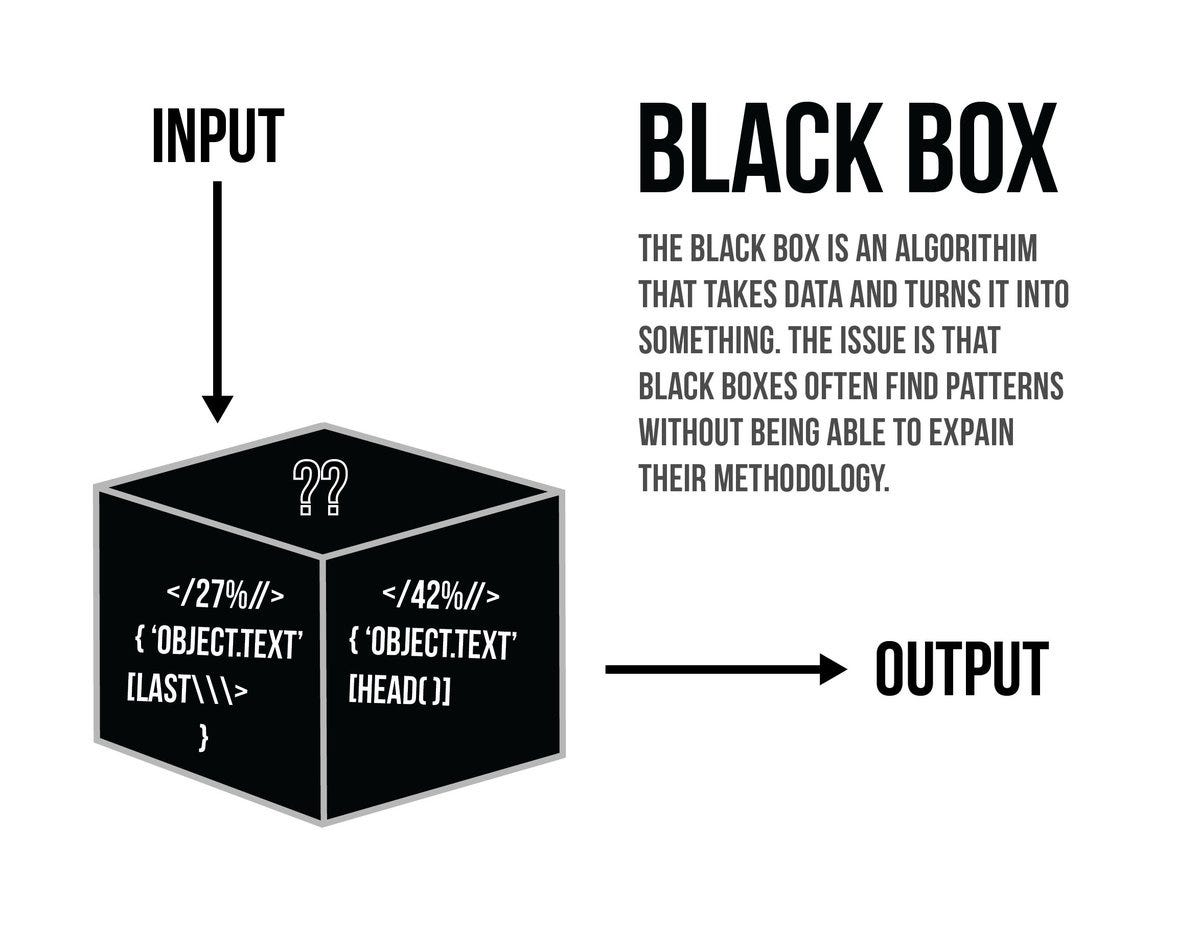The black box algorithm — who knows what it’s doing? Apparently, nobody.

Accuracy alone is no longer enough.

Academics in deep learning are acutely aware of this interpretability and explainability problem, and whilst some argue (such as Sam Harris in the above quote) that these models are essentially black boxes, there have been several developments in recent years which have been developed for visualizing aspects of deep neural networks such the features and representations they have learned. The term info-besity has been thrown around to refer to the difficulty of providing transparency when decisions are made on the basis of many individual features, due to an overload of information. The field of interpretability and explainability in machine learning has exploded since 2015 and there are now dozens of papers on the subject, some of which can be found in the references.

As we will see in this article, these visualization techniques are not sufficient for completely explaining the complex representations learned by deep learning algorithms, but hopefully, you will be convinced that the black box interpretation of deep learning is not true — we just need better techniques to be able to understand and interpret these models.

# The Black Box

All algorithms in machine learning are to some extent black boxes. One of the key ideas of machine learning is that the models are data-driven — the model is configured from the data. This fundamentally leads us to problems such as (1) how we should interpret the models, (2) how to ensure they are transparent in their decision making, and (3) making sure the results of the said algorithm are fair and statistically valid.

For something like linear regression, the models are very well understood and highly interpretable. When we move to something like a support vector machine (SVM) or a random forest model, things get a bit more difficult. In this sense, there is no white or black box algorithm in machine learning, the interpretability exists as a spectrum or a ‘gray box’ of varying grayness.

It just so happens, that at the far end of our ‘gray’ area is the neural network. Even further in this gray area is the deep neural network. When you have a deep neural network with 1.5 billion parameters — as the GPT-2 algorithm for language modeling has — it becomes extremely difficult to interpret the representations that the model has learned.

In February 2020, Microsoft released the largest deep neural network in existence (probably not for long), Turing-NLG. This network contains 17 billion parameters, which is around 1/5th of the 85 billion neurons present in the human brain (although in a neural network, parameters represent connections, of which there are ~100 trillion in the human brain). Clearly, interpreting a 17 billion parameter neural network will be incredibly difficult, but its performance may be far superior to other models because it can be trained on huge amounts of data without becoming saturated — this is the idea that more complex representations can be stored by a model with a greater number of parameters.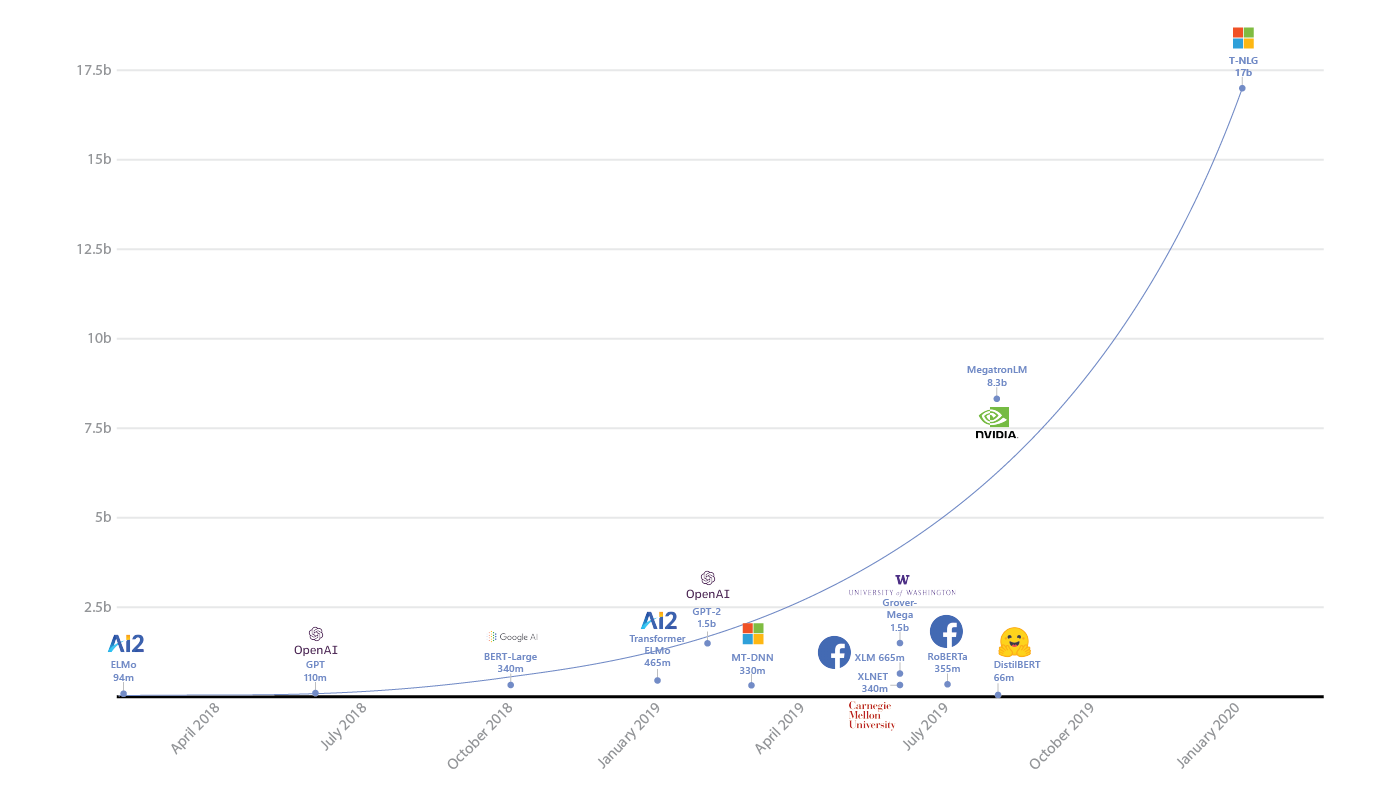Comparison of Turing-NLG to other deep neural networks such as BERT and GPT-2. Source

Obviously, the representations are there, we just do not understand them fully, and thus we must come up with better techniques to be able to interpret the models. Sadly, it is more difficult than reading coefficients as one is able to do in linear regression!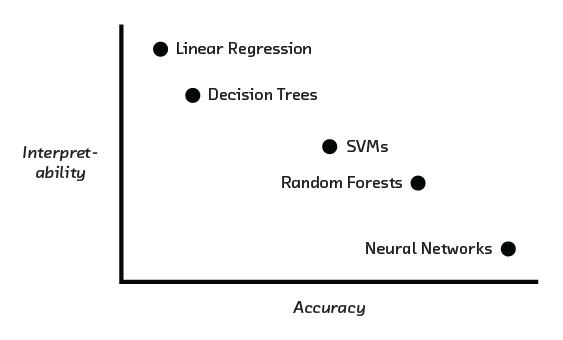Neural networks are powerful models, but harder to interpret than simpler and more traditional models.

Often, we do not care how an algorithm came to a specific decision, particularly when they are operationalized in low-risk environments. In these scenarios, we are not limited in our selection of algorithms by any limitation on the interpretability. However, if interpretability is important within our algorithm — as it often is for high-risk environments — then we must accept a tradeoff between accuracy and interpretability.

So what techniques are available to help us better interpret and understand our models? It turns out there are many of these, and it is helpful to make a distinction between what these different types of techniques help us to examine.

Local vs. Global

Techniques can be local, to help us study a small portion of the network, as is the case when looking at individual filters in a neural network.

Techniques can be global, allowing us to build up a better picture of the model as a whole, this could include visualizations of the weight distributions in a deep neural network, or visualizations of neural network layers propagating through the network.

Model-Specific vs. Model-Agnostic

A technique that is highly model-specific is only suitable for use by a single type of models. For example, layer visualization is only applicable to neural networks, whereas partial dependency plots can be utilized for many different types of models and would be described as model-agnostic.

Model-specific techniques generally involve examining the structure of algorithms or intermediate representations, whereas model-agnostic techniques generally involve examining the input or output data distribution.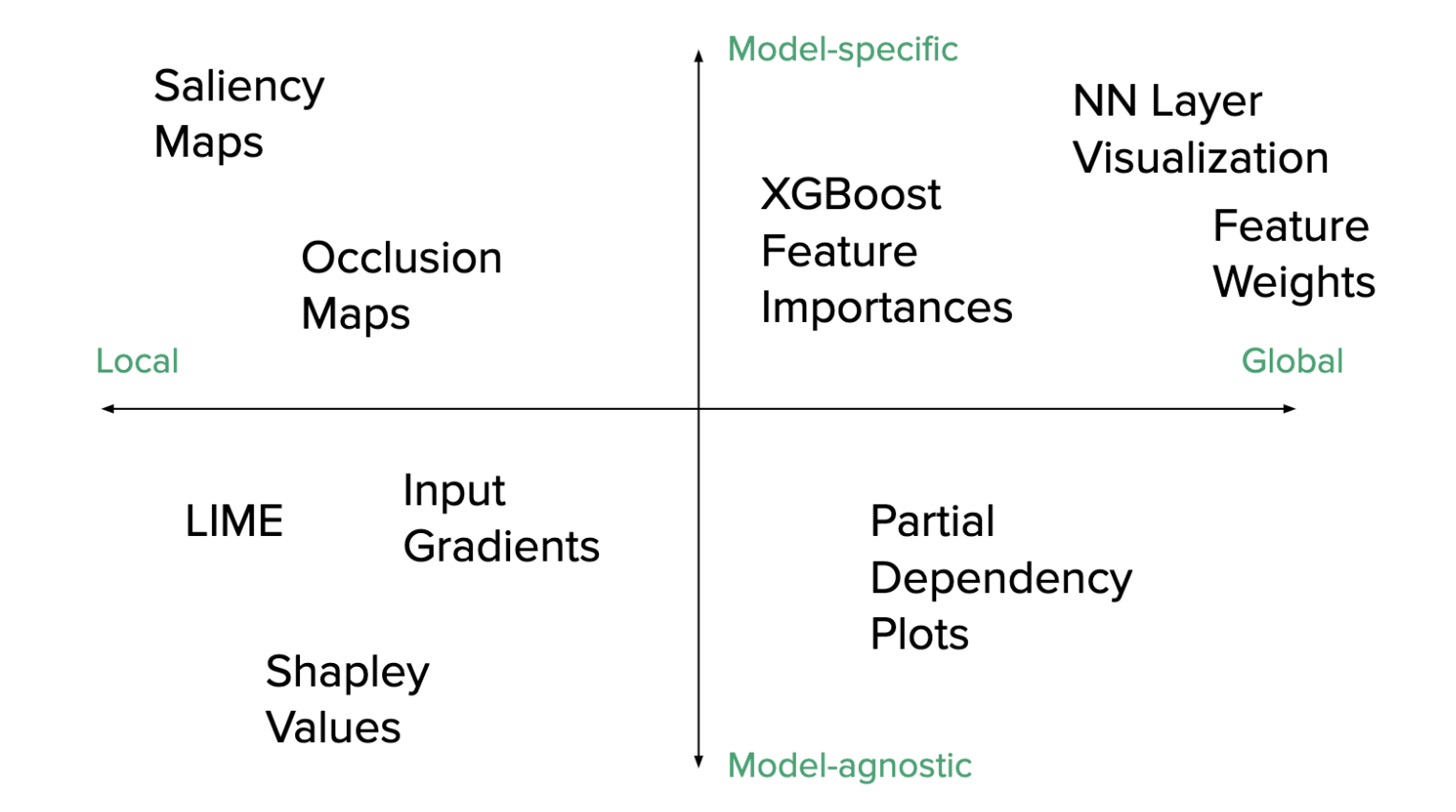The distinction between different model visualization techniques and interpretability metrics. Source

I will discuss all of the above techniques throughout this article, but will also discuss where and how they can be put to use to help provide us with insight into our models.

Being Right for the Right Reasons

One of the issues that arise from our lack of model explainability is that we do not know what the model has been trained on. This is best illustrated with an apocryphal example (there is some debate as to the truth of the story, but the lessons we can draw from it are nonetheless valuable).

Hide and Seek

According to AI folklore, in the 1960s, the U.S. Army was interested in developing a neural network algorithm that was able to detect tanks in images. Researchers developed an algorithm that was able to do this with remarkable accuracy, and everyone was pretty happy with the result.

However, when the algorithm was tested on additional images, it performed very poorly. This confused the researchers as the results had been so positive during development. After a while of everyone scratching their heads, one of the researchers noticed that when looking at the two sets of images, the sky was darker in one set of images than the other.

It became clear that the algorithm had not actually learned to detect tanks that were camouflaged, but instead was looking at the brightness of the sky!

Whilst this story exacerbates one of the common criticisms of deep learning, there is truth to the fact that in a neural network, and especially a deep neural network, you do not really know what the model is learning.

This powerful criticism and the increasing importance of deep learning in academia and industry is what has led to an increased focus on interpretability and explainability. If an industry professional cannot convince their client that they understand what the model they built is doing, should it be really be used when there are large risks, such as financial losses or people’s lives?

## Interpretability

At this point, you might be asking yourself how visualization can help us to interpret a model, given that there may be an infinite number of viable interpretations. Defining and measuring what interpretability means is not a trivial task, and there is little consensus on how to evaluate it.

There is no mathematical definition of interpretability. Two proposed definitions in the literature are:

“Interpretability is the degree to which a human can understand the cause of a decision.”** — Tim Miller**

“Interpretability is the degree to which a human can consistently predict the model’s result.” — Been Kim

The higher the interpretability of a machine learning model, the easier it is for someone to comprehend why certain decisions or predictions have been made. A model is better interpretable than another model if its decisions are easier for a human to comprehend than decisions from the other model. One way we can start to evaluate model interpretability is via a quantifiable proxy.

proxy is something that is highly correlated with what we are interested in studying but is fundamentally different from the object of interest. Proxies tend to be simpler to measure than the object of interest, or like in this case, just measurable — whereas our object of interest (like interpretability) may not be.

The idea of proxies is prevalent in many fields, one of which is psychology where they are used to measure abstract concepts. The most famous proxy is probably the intelligence quotient (IQ) which is a proxy for intelligence. Whilst the correlation between IQ and intelligence is not 100%, it is high enough that we can gain some useful information about intelligence from measuring IQ. There is no known way for directly measuring intelligence.

An algorithm that uses dimensional reduction to allow us to visualize high-dimensional data in a lower-dimensional space provides us with a proxy to visualize the data distribution. Similarly, a set of training images provides us with a proxy of the full data distribution of interest, but will inevitably be somewhat different to the true distribution (if you did a good job constructing the training set, it should not differ too much from a given test set).

Post-hoc explanations (or explaining after the fact) can be useful but sometimes misleading. These merely provide a plausible rationalization for the algorithmic behavior of a black box, not necessarily concrete evidence and so should be used cautiously. Post-hoc rationalization can be done with quantifiable proxies, and some of the techniques we will discuss do this.

# Choosing a Visualization

Designing a visualization requires us to think about the following factors:

• **The audience to whom we are presenting (the who) **— is this being done for debugging purposes? To convince a client? To convince a peer-reviewer for a research article?
• The objective of the visualization (the what)— are we trying to understand the inputs (such as if EXIF metadata from an image is being read correctly so that an image does not enter a CNN sideways), outputs, or parameter distributions of our model? Are we interested in how inputs evolve through the network or a static feature of the network like a feature map or filter?
• The model being developed (the how)— clearly, if you are not using a neural network, you cannot visualize feature maps of a network layer. Similarly, feature importance can be used for some models, such as XGBoost or Random Forest algorithms, but not others. Thus the model selection inherently biases what techniques can be used, and some techniques are more general and versatile than others. Developing multiple models can provide more versatility in what can be examined.

Deep models present unique challenges for visualization: we can answer the same questions about the model, but our method of interrogation must change! Because of the importance of this, we will mainly focus on deep learning visualization for the rest of the article.

## Subfields of Deep Learning Visualization

There are largely three subfields of deep learning visualization literature:

1. Interpretability & Explainability: helping to understand how deep learning models make decisions and their learned representations.
2. Debugging & Improving: helping model curators and developers construct and troubleshoot their models, with the hope of expediting the iterative experimentation process to ultimately improve performance.
3. Teaching Deep Learning: helping to educate amateur users about artificial intelligence — more specifically, machine learning.

## Why is interpreting a neural network so difficult?

To understand why interpreting a neural network is difficult and non-intuitive, we have to understand what the network is doing to our data.

Essentially, the data we pass to the input layer — this could be an image or a set of relevant features for predicting a variable — can be plotted to form some complex distribution like that shown in the image below (this is only a 2D representation, imagine it in 1000 dimensions).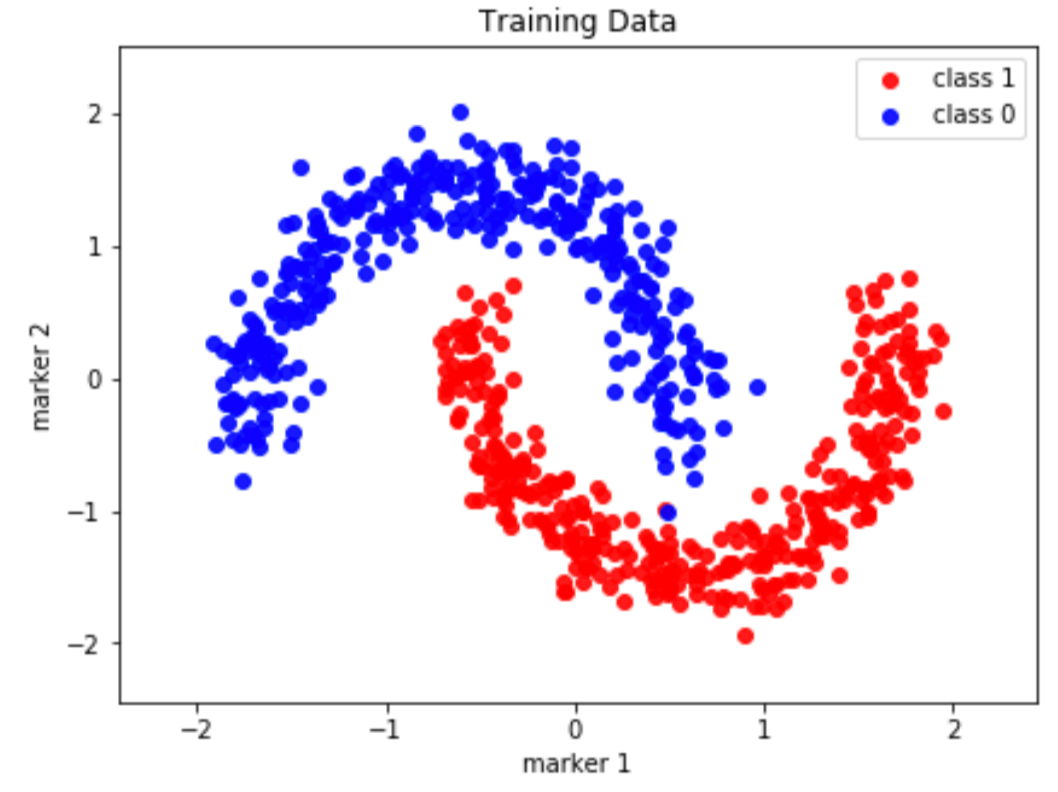If we ran this data through a linear classifier, the model would try its best to separate the data, but since we are limited to a hypothesis class that only contains linear functions, our model will perform poorly since a large portion of the data is not linearly separable.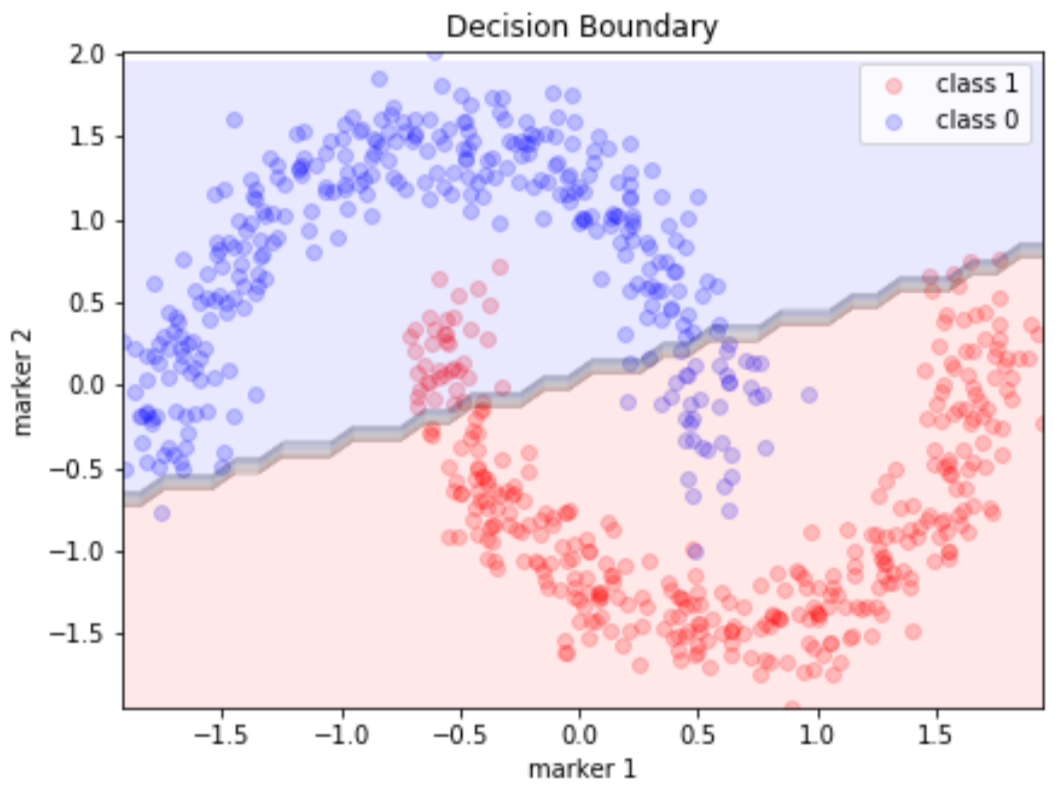This is where neural networks come in. The neural network is a very special function. It has been proven that a neural network with a single hidden layer is capable of representing the hypothesis class of all non-linear functions, as long as we have enough nodes in the network. This is known as the universal approximation theorem.

It turns out that the more nodes we have, the larger our class of functions we can represent. If we have a network with only ten layers and are trying to use it to classify a million images, the network will quickly saturate and reach maximum capacity. If we have 10 million parameters, it will be able to learn a much better representation of the network, as the number of non-linear transformations increases. We say this model has a larger model capacity.

People use deep neural networks instead of a single layer because the amount of neurons needed in a single layer network increases exponentially with model capacity. The abstraction of hidden layers significantly reduces the need for more neurons but this comes at a cost for interpretability. The deeper we go, the less interpretable the network becomes.

The non-linear transformations of the neural network allow us to remap our data into a linearly separable space. At the output layer of a neural network, it then becomes arbitrary for us to separate our initially non-linear data into two classes using a linear classifier, as illustrated below.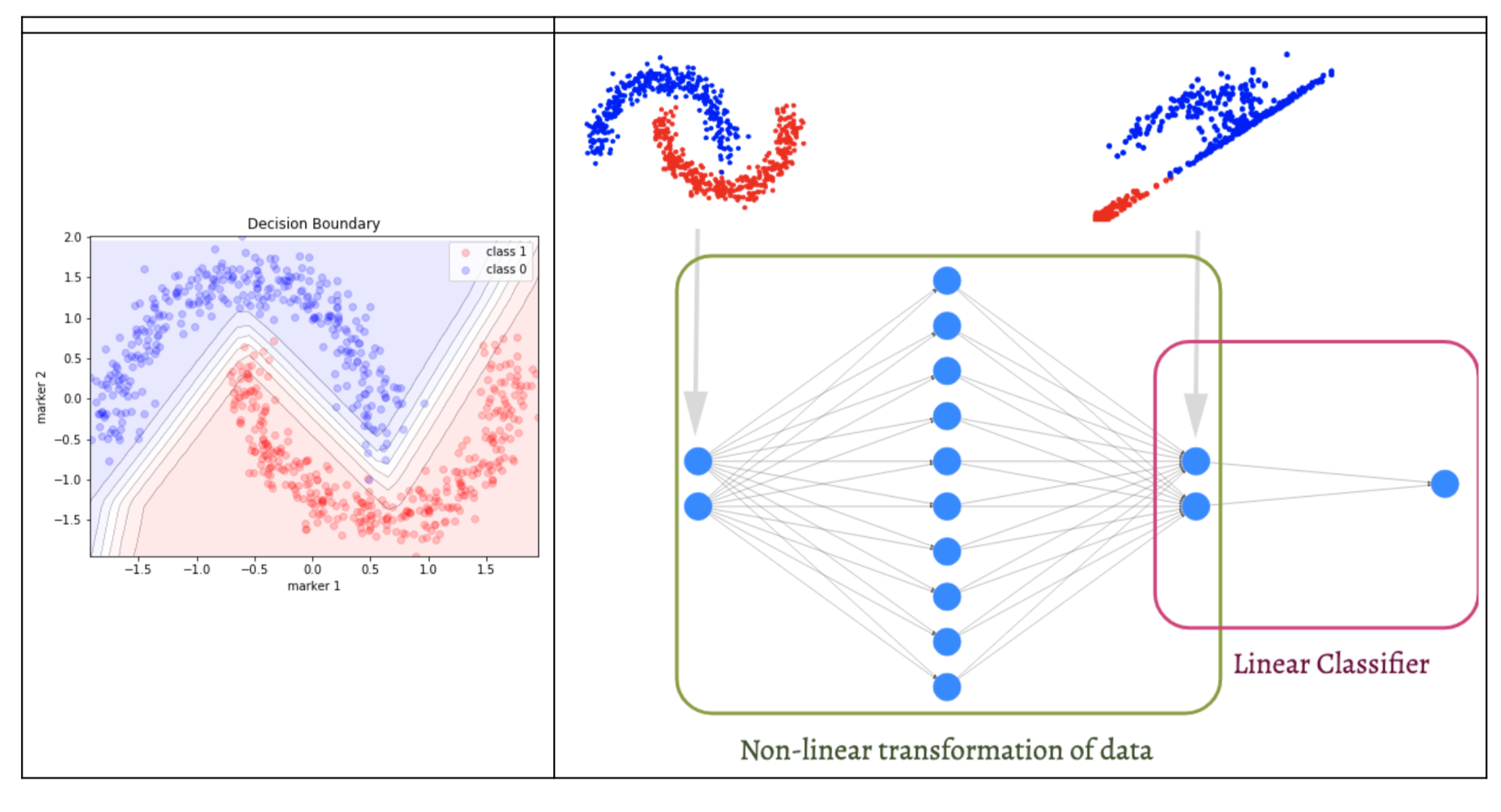The transformation of a non-linear dataset to one that is linearly separable using a neural network. Source

The question is, how do we know what is going on within this multi-layer non-linear transformation, which may contain millions of parameters?

Imagine a GAN model (two networks fighting each other in order to mimic the distribution of the input data) working on a 512×512 image dataset. When images are introduced into a neural network, each pixel becomes a feature of the neural network. For an image of this size, the number of features is 262,144. This means we are performing potentially 8 or 9 convolutional and non-linear transformations on over 200,000 features. How can one interpret this?

Go even more extreme to the case of 1024×1024 images, which have been developed by NVIDIA’s implementation of StyleGAN. Since the number of pixels increases by a factor of four with a doubling of image size, we would have over a million features as our input to the GAN. So we now have a one million feature neural network, performing convolutional operations and non-linear activations, and doing this over a dataset of hundreds of thousands of images.

Hopefully, I have convinced you that interpreting deep neural networks is profoundly difficult. Although the operations of a neural network may seem simple, they can produce wildly complex outcomes via some form of emergence.

#ai & machine learning #algorithm #black box #deep learning #machine learning #deep learning1597118580

## What is Machine learning and Why is it Important?

Machine learning is quite an exciting field to study and rightly so. It is all around us in this modern world. From Facebook’s feed to Google Maps for navigation, machine learning finds its application in almost every aspect of our lives.

It is quite frightening and interesting to think of how our lives would have been without the use of machine learning. That is why it becomes quite important to understand what is machine learning, its applications and importance.

But before we answer these questions, it is important to first know about the history of machine learning.

### A Brief History of Machine Learning

You might think that machine learning is a relatively new topic, but no, the concept of machine learning came into the picture in 1950, when Alan Turing (Yes, the one from Imitation Game) published a paper answering the question “Can machines think?”.

In 1957, Frank Rosenblatt designed the first neural network for computers, which is now commonly called the Perceptron Model.In 1959, Bernard Widrow and Marcian Hoff created two neural network models called Adeline, that could detect binary patterns and Madeline, that could eliminate echo on phone lines.

In 1967, the Nearest Neighbor Algorithm was written that allowed computers to use very basic pattern recognition.

Gerald DeJonge in 1981 introduced the concept of explanation-based learning, in which a computer analyses data and creates a general rule to discard unimportant information.

During the 1990s, work on machine learning shifted from a knowledge-driven approach to a more data-driven approach. During this period, scientists began creating programs for computers to analyse large amounts of data and draw conclusions or “learn” from the results. Which finally overtime after several developments formulated into the modern age of machine learning.

Now that we know about the origin and history of ml, let us start by answering a simple question - What is Machine Learning?

#machine-learning #machine-learning-uses #what-is-ml #supervised-learning #unsupervised-learning #reinforcement-learning #artificial-intelligence #ai1601344800

## Machine Learning | Everything you need to know

Machine learning is enabling computers to tackle tasks that have, until now, only been carried out by people.

From driving cars to translating speech, machine learning is driving an explosion in the capabilities of artificial intelligence— helping software make sense of the messy and unpredictable real world.

But what exactly is machine learning and what is making the current boom in machine learning possible?

#supervised-learning #machine-learning #reinforcement-learning #semi-supervised-learning #unsupervised-learning1617750180

## Supervised and Unsupervised learning — simplified

A discussion on popular approaches — supervised, unsupervised and reinforcement learning.
Machine Learning algorithms are a set of algorithms that simulate learning behaviour in computing systems. These algorithms learn patterns from data which can then be used to predict or infer new knowledge from new unseen data. There are many approaches to machine learning. The most popular ones are classed as supervised, unsupervised and reinforcement learning.

#reinforcement-learning #machine-learning #unsupervised-learning #supervised-learning #ai